﻿ 深空网测控模式△DOR测量建模与精度分析
«上一篇文章快速检索 高级检索

 力学学报2015, Vol. 47Issue (1): 24-30  DOI: 10.6052/0459-1879-14-3280

### 引用本文 [复制中英文]

[复制中文]
Tang Geshi, Han Songtao, Cao Jianfeng, Chen Lüe, Ren Tianpeng, Wang Mei. MODEL AND ANALYSIS OF ΔDOR TRACKING BY CHINA DSN WITH TT&C MODE[J]. Chinese Journal of Ship Research, 2015, 47(1): 24-30. DOI: 10.6052/0459-1879-14-328.
[复制英文]

### 文章历史

2014-10-23 收稿
2014-11-27 录用
2014–12–12 网络版发表

1. 航天飞行动力学技术重点实验室, 北京 100094;
2. 北京航天飞行控制中心, 北京 100094

1 差分干涉测量原理简介[13, 14, 15]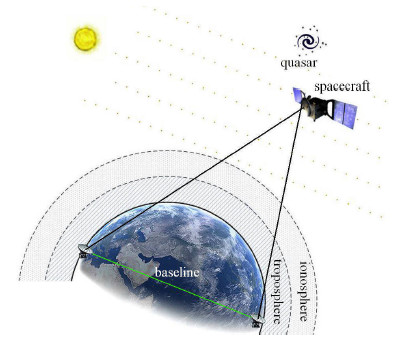图 1 $\vartriangle$DOR测量基本原理图 Fig. 1 Theory of $\vartriangle$DOR tracking

 $\tau _{SC} = \tau _{g\_SC} + \Delta \tau _{SC} =\\ \qquad \tau _{g\_SC} + \tau _{{\rm clock}\_SC} + \tau_{{\rm atm}\_SC} + \tau _{{\rm path}\_SC}$ (1)

 $\tau _{\rm egrs} = \tau _{g\_{\rm egrs}} + \Delta \tau _{\rm egrs} =\\ \qquad \tau _{g\_{\rm egrs}} + \tau _{\rm clock\_egrs} + \tau_{\rm atm\_egrs} + \tau _{\rm path\_egrs}$ (2)

 $\Delta \tau _{\rm egrs} = \tau _{\rm egrs} - \tau _{g\_{\rm egrs} }$ (3)

2 测控模式差分干涉测量技术建模

2.1 站间时间同步测量

 $\tau _{{\rm clock}\_SC} = k_1 (t - t_0 ) + k_0$ (4)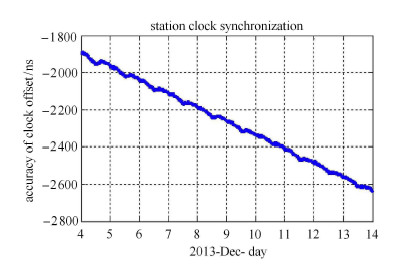图 2 站间时间同步精度分析 Fig. 2 Analysis of station clock synchronization

 $\Delta_{\Delta { \tau}_{SC}} = ( 1 \ \ 1 \ \ 1) \left( \!\!\begin{array}{c} {\Delta \tau _{{\rm clock}\_SC} } \\ {\Delta \tau _{{\rm med}\_SC} } \\ {\Delta \tau _{{\rm path}\_SC} } \end{array} \!\! \right)$ (5)

 $\Delta_{\Delta \tau _{SC}} = \Delta k_1 (t - t_0 ) + \Delta k_0$ (6)

2.2 传播介质时延误差修正

 $\Delta S = \dfrac{K_d }{\sin (E^2 + 6.25)^{1 / 2}} + \dfrac{K_w }{\sin (E^2 + 2.25)^{1 / 2}}$ (7)

2.3 设备链路时延误差修正

 $x_{\rm pcal} (t) = A\exp ( {\rm i}2\pi f_{\rm pcal} (t - \tau _{\rm path} ))$ (8)

 $\begin{array}{l} \Phi = {\rm{angle}}(\int {{x_{{\rm{pcal}}}}(t) \cdot {\rm{conj}}\left\{ {{x_{{\rm{ref}}}}(t)} \right\}{\rm{d}}t} ) = \\ \qquad - 2\pi {f_{{\rm{pcal}}}}{\tau _{{\rm{path}}}} \end{array}$ (9)

2.4 射电源稀疏标校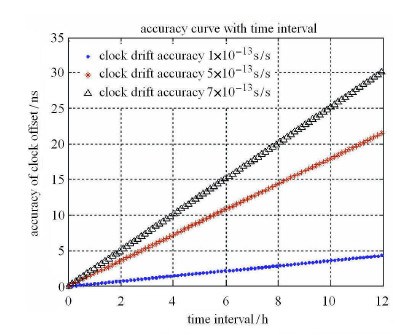图 3 误差修正精度与观测时间间隔的关系图 Fig. 3 Accuracy curve with time interval

3 精度分析与验证

CE$'$3号于2013年12月2日发射，12月6日进入100 km$\times$100 km环月轨道，12月10日降轨至100 km$\times$15 km轨道. CE$'$3号在轨运行期间，中国深空网佳木斯深空站、喀什深空站负责CE$'$3号的测控任务. 实验观测选择2013年12月13日北京时间19:00$\sim$23:10，在该时段内CVN (China VLBI Net)网同期进行了CE$'$3号传统短时交替模式的差分干涉测量. 下表列出了本次实验深空网的具体观测信息及采集参数.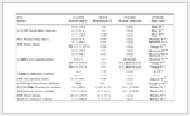表 1 系统参数 Table 1 System parameters

3.1 数据处理结果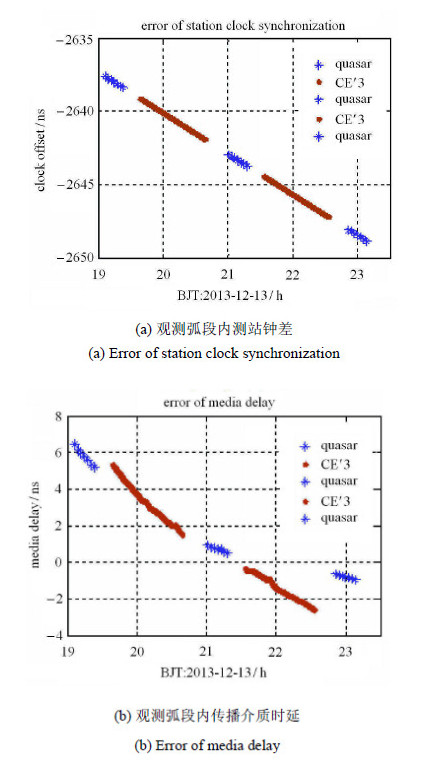图 4 观测弧段内钟差与传播介质时延分析 Fig. 4 Error of clock synchronization and media delay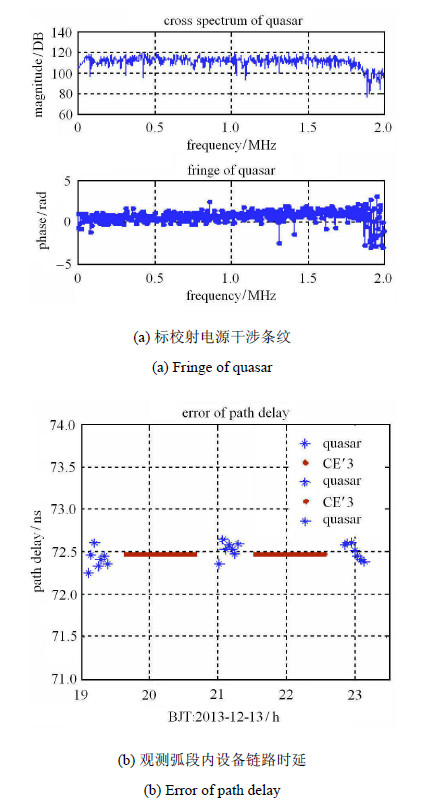图 5 观测弧段内射电源干涉条纹与链路时延分析 Fig. 5 Fringe of quasar and path delay

CE$'$3号干涉测量信号体制为相干转发模式，主载波上调制有遥测信息，两组DOR侧音信号与主载波相干. 图6显示了航天器主 载波通道的互相关谱以及相关计算时延观测量.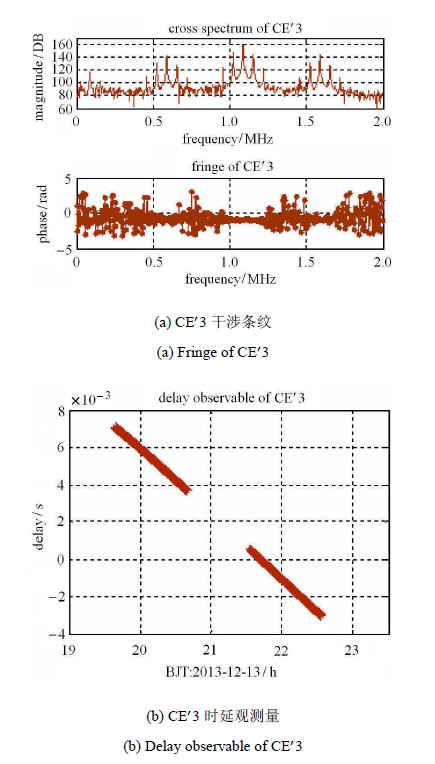图 6 观测弧段内CE$'$3干涉条纹与观测量 Fig. 6 Fringe of CE$'$3 and delay observable
3.2 精度比对分析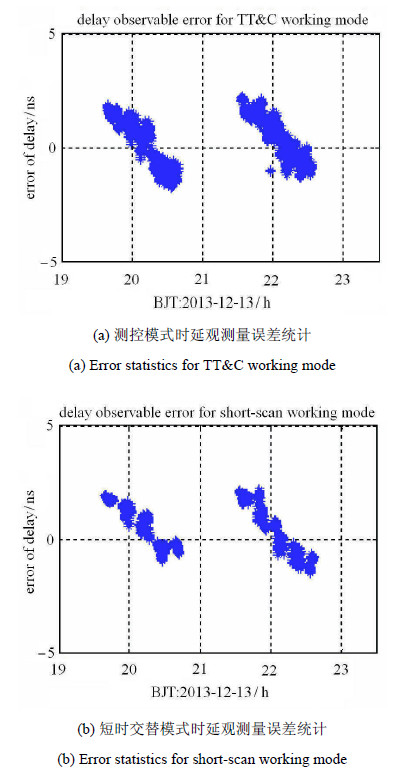图 7 观测量误差统计 Fig. 7 Error statistics of delay observable

4 结 论

Tang Geshi, Han Songtao, Cao Jianfeng, Chen Lüe, Ren Tianpeng, Wang Mei